# 1 Introduction to fCI

## 1.1 Authors and Affliations

Shaojun Tang1, Martin Hemberg2, Ertugrul Cansizoglu3, Stephane Belin3, Kenneth Kosik4, Gabriel Kreiman2, Hanno Steen1#+, Judith Steen3#+

1Departments of Pathology, Boston Childrens Hospital and Harvard Medical School, Boston, MA, USA, 02115

2Department of Ophthalmology, Boston Childrens Hospital, Boston, MA, USA, 02115

3F.M. Kirby Neurobiology Center, Childrens Hospital, and Department of Neurology, Harvard Medical School, 300 Longwood Avenue, Boston, MA, USA, 02115

4Neuroscience Research Institute, University of California at Santa Barbara, Santa Barbara, CA, USA, 93106"

## 1.2 Abstract

“The ability to integrate ‘omics’ (i.e., transcriptomics and proteomics) is becoming increasingly important to understanding regulatory mechanisms. There are currently no tools available to identify differentially expressed genes (DEGs) across different ‘omics’ data types or multi-dimensional data including time courses. We present a model capable of simultaneously identifying DEGs from continuous and discrete transcriptomic, proteomic and integrated proteogenomic data. We show that our algorithm can be used across multiple diverse sets of data and can unambiguously find genes that show functional modulation, developmental changes or misregulation. Applying our model to a time course proteogenomics dataset, we identified a number of important genes that showed distinctive regulation patterns.

## 1.3 Introduction

fCI (f-divergence Cutoff Index), identifies DEGs by computing the difference between the distribution of fold-changes for the control-control and remaining (non-differential) case-control gene expression ratio data.As a null hypothesis, we assume that the control samples, regardless of data types, do not contain DEGs and that the spread of the control data reflects the biological and technical variance in the data. In contrast, the case samples contain a yet unknown number of DEGs. Removing DEGs from the case data leaves a set of non-differentially expressed genes whose distribution is identical to the control samples. Our method, f-divergence cut-out index (fCI) identifies DEGs by computing the difference between the distribution of fold-changes for the control-control data and remaining (non-differential) case-control gene expression ratio data (see Fig. 1.a-b) upon removal of genes with large fold changes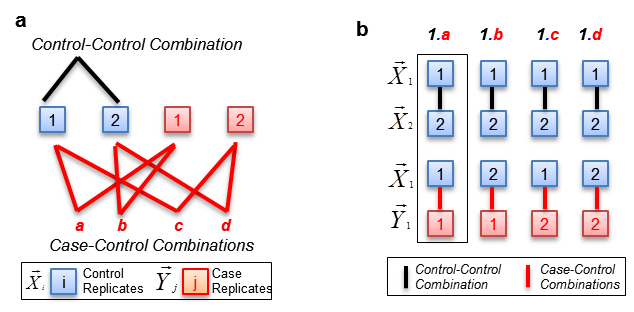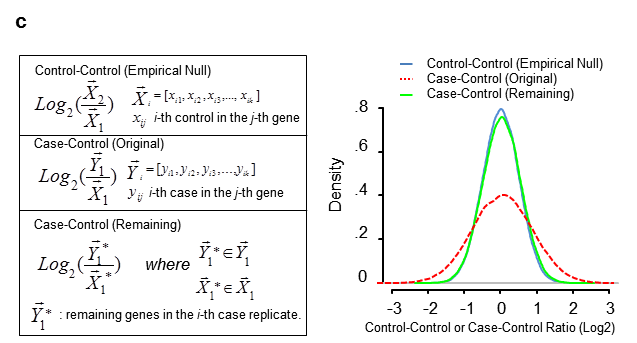fCI provides several advantages compared to existing methods. Firstly, it performed equally well or better in finding DEGs in diverse data types (both discrete and continuous data) from various omics technologies compared to methods that were specifically designed for the experiments. Secondly, it fulfills an urgent need in the omics research arena. The increasingly common proteogenomic approaches enabled by rapidly decreasing sequencing costs facilitates the collection of multi-dimensional (i.e. proteogenomics) experiments, for which no efficient tools have been developed to find co-regulation and dependences of DEGs between treatment conditions or developmental stages. Thirdly, fCI does not rely on statistical methods that require sufficiently large numbers of replicates to evaluate DEGs. Instead fCI can effectively identify changes in samples with very few or no replicates.

# 2 Installing fCI

fCI should be installed as follows:

source("http://bioconductor.org/biocLite.R")
biocLite("fCI")
 suppressPackageStartupMessages(library(fCI))
library(fCI)

# 3 Differential Expression Analysis using fCI

fCI is very usefriendly. Users only need to provide a ‘Tab’ delimited input data file and give the indexes of control and case samples.

## 3.1 Reading the input data:

Read Inupt Data to R** . This input will contain gene, protein or other expression values with columns representing samples/lanes/replicates, and rows representing genes.

### 3.1.1 Integer raw read counts from NGS data or Spectrum counts from proteomics data

As input, the fCI package could analysis count data as obtained, e. g., from RNA-seq or another high-throughput sequencing experiment, in the form of a matrix of integer values. The value in the i-th row and the j-th column of the matrix tells how many reads have been mapped to gene i in sample j. Analogously, for other types of assays, the rows of the matrix might correspond e. g. to binding regions (with ChIP-Seq) or peptide sequences (with quantitative mass spectrometry).

### 3.1.2 Normalized gene expression such as RPKM or FPKM, or peak intesntiy (height/area) in proteomics data

The fCI package could also analyze decimal data in the form of RPKM/FPKM from RNA-seq or another high-throughput sequencing experiment, in the form of a matrix of integer values. The value in the i-th row and the j-th column of the matrix tells the normalized expression level in gene i and sample j.

### 3.1.3 Ratio data from many experiments measuring relative gene expression with respect to control channels.

For example, relative protein quantification by MS/MS using the tandem mass tag technology are represented by ratios.

## 3.2 Data normalization

### 3.2.1 Total library normalization

The samples are normalized to have the same library size (i.e. total raw read counts) if the experiment replicates were obtained by the same protocol and an equal library size was expected within each experimental condition. The fCI will apply the sum normalization so that each column has equal value by summing all the genes of each replicate.

fci.data=data.frame(matrix(sample(3:100, 1043*6, replace=TRUE), 1043,6))
fci.data=total.library.size.normalization(fci.data)

### 3.2.2 Trimed sum normalization

We could normalize each replicate to have the same library size (total read count) after the 5% lowly expressed and the 5% highly expressed genes were removed from each replicate

fci.data=data.frame(matrix(sample(3:100, 1043*6, replace=TRUE), 1043,6))
fci.data=trim.size.normalization(fci.data)

### 3.2.3 Kernel density distribution centering

We hypothesized that the genes whose expression was the least affected by the experiment (in the forms of both RNA and protein) should have nearly identical expression levels across different replicates, in both RNA-Seq and proteomic datasets. These unchanged genes will be centered at zero in the logarithm transformed control-control or case-control ratio distributions. Therefore, we normalized proteogenomic dataset’s fCI pairwise ratio distribution (Gaussian kernel density approximation) to be centered at zero.

## 3.3 fCI analysis with the Spike-in microarray data

• The Spike-in data contained a number of spiked-in differentially expressed genes with a known cutoff of 1.4 fold threshold.

• The input data is a tab-delimited file with rows representing genes and columns being the samples of control and experimental treatments. T

• To find the DEGs, we first created a fCI class object named fci, which will be passed onto the main function “find.fci.targets”. In the function call, the users need to specify the control sample column ids (such as a vector of 1, 2 and 3) and case sample column ids (such as a vector of 4, 5 and 6). Each sample must contain the same number of genes.

• For the chosen control samples, fCI forms a list of the control-control combinations, namely 1-2, 1-3 and 2-3, each containing two unique replicates from the full set of control replicates. Similarly, fCI forms a list of control-case combinations, namely, 1-4, 1-5, 1-6, 2-4, 2-5, 2-6, 3-4, 3-5 and 3-6, each containing a unique replicate from the control and a unique replicate from the case samples.

pkg.path=path.package('fCI')
filename=paste(pkg.path, "/extdata/Supp_Dataset_part_2.txt", sep="")

if(file.exists(filename)){
fci=new("NPCI")
fci=find.fci.targets(fci, c(1,2,3), c(4,5,6), filename)
}
## Control-Control Used : [ 1 2 ] & Control-Case Used : [ 1 4 ];  Fold_Cutoff= 1.3 ; Num_Of_DEGs= 821 ; Divergence= 0.00015769
## Control-Control Used : [ 1 2 ] & Control-Case Used : [ 2 4 ];  Fold_Cutoff= 1.3 ; Num_Of_DEGs= 811 ; Divergence= 0.00069831
## Control-Control Used : [ 1 2 ] & Control-Case Used : [ 3 4 ];  Fold_Cutoff= 1.3 ; Num_Of_DEGs= 813 ; Divergence= 0.00021425
## Control-Control Used : [ 1 2 ] & Control-Case Used : [ 1 5 ];  Fold_Cutoff= 1.3 ; Num_Of_DEGs= 820 ; Divergence= 0.00056408
## Control-Control Used : [ 1 2 ] & Control-Case Used : [ 2 5 ];  Fold_Cutoff= 1.3 ; Num_Of_DEGs= 819 ; Divergence= 0.00010632
## Control-Control Used : [ 1 2 ] & Control-Case Used : [ 3 5 ];  Fold_Cutoff= 1.3 ; Num_Of_DEGs= 820 ; Divergence= 0.00057505
## Control-Control Used : [ 1 2 ] & Control-Case Used : [ 1 6 ];  Fold_Cutoff= 1.3 ; Num_Of_DEGs= 823 ; Divergence= 0.00023359
## Control-Control Used : [ 1 2 ] & Control-Case Used : [ 2 6 ];  Fold_Cutoff= 1.3 ; Num_Of_DEGs= 813 ; Divergence= 0.00113844
## Control-Control Used : [ 1 2 ] & Control-Case Used : [ 3 6 ];  Fold_Cutoff= 1.3 ; Num_Of_DEGs= 813 ; Divergence= 0.00036187
## Control-Control Used : [ 1 3 ] & Control-Case Used : [ 1 4 ];  Fold_Cutoff= 1.3 ; Num_Of_DEGs= 821 ; Divergence= 1.764e-05
## Control-Control Used : [ 1 3 ] & Control-Case Used : [ 2 4 ];  Fold_Cutoff= 1.3 ; Num_Of_DEGs= 811 ; Divergence= 0.00014706
## Control-Control Used : [ 1 3 ] & Control-Case Used : [ 3 4 ];  Fold_Cutoff= 1.3 ; Num_Of_DEGs= 813 ; Divergence= 1.477e-05
## Control-Control Used : [ 1 3 ] & Control-Case Used : [ 1 5 ];  Fold_Cutoff= 1.3 ; Num_Of_DEGs= 820 ; Divergence= 0.00014493
## Control-Control Used : [ 1 3 ] & Control-Case Used : [ 2 5 ];  Fold_Cutoff= 1.3 ; Num_Of_DEGs= 819 ; Divergence= 2.89e-06
## Control-Control Used : [ 1 3 ] & Control-Case Used : [ 3 5 ];  Fold_Cutoff= 1.3 ; Num_Of_DEGs= 820 ; Divergence= 0.00012726
## Control-Control Used : [ 1 3 ] & Control-Case Used : [ 1 6 ];  Fold_Cutoff= 1.3 ; Num_Of_DEGs= 823 ; Divergence= 5.034e-05
## Control-Control Used : [ 1 3 ] & Control-Case Used : [ 2 6 ];  Fold_Cutoff= 1.3 ; Num_Of_DEGs= 813 ; Divergence= 0.00024359
## Control-Control Used : [ 1 3 ] & Control-Case Used : [ 3 6 ];  Fold_Cutoff= 1.3 ; Num_Of_DEGs= 813 ; Divergence= 3.525e-05
## Control-Control Used : [ 2 3 ] & Control-Case Used : [ 1 4 ];  Fold_Cutoff= 1.3 ; Num_Of_DEGs= 821 ; Divergence= 2e-08
## Control-Control Used : [ 2 3 ] & Control-Case Used : [ 2 4 ];  Fold_Cutoff= 1.3 ; Num_Of_DEGs= 811 ; Divergence= 1.509e-05
## Control-Control Used : [ 2 3 ] & Control-Case Used : [ 3 4 ];  Fold_Cutoff= 1.3 ; Num_Of_DEGs= 813 ; Divergence= 2.82e-06
## Control-Control Used : [ 2 3 ] & Control-Case Used : [ 1 5 ];  Fold_Cutoff= 1.3 ; Num_Of_DEGs= 820 ; Divergence= 8.66e-06
## Control-Control Used : [ 2 3 ] & Control-Case Used : [ 2 5 ];  Fold_Cutoff= 1.3 ; Num_Of_DEGs= 819 ; Divergence= 2.82e-06
## Control-Control Used : [ 2 3 ] & Control-Case Used : [ 3 5 ];  Fold_Cutoff= 1.3 ; Num_Of_DEGs= 820 ; Divergence= 8.36e-06
## Control-Control Used : [ 2 3 ] & Control-Case Used : [ 1 6 ];  Fold_Cutoff= 1.3 ; Num_Of_DEGs= 823 ; Divergence= 5.8e-07
## Control-Control Used : [ 2 3 ] & Control-Case Used : [ 2 6 ];  Fold_Cutoff= 1.3 ; Num_Of_DEGs= 813 ; Divergence= 6.742e-05
## Control-Control Used : [ 2 3 ] & Control-Case Used : [ 3 6 ];  Fold_Cutoff= 1.3 ; Num_Of_DEGs= 813 ; Divergence= 1.48e-05

## 3.4 fCI DEG analysis Output

• the returned object ‘fci’ will contains all the differentially expressed genes and runtime variables, including the DEGs and kernel density distributions.

### 3.4.2 The Kernel Density Plot of Control-Control and Control-Case distributions

  figures(fci)
##  426.0178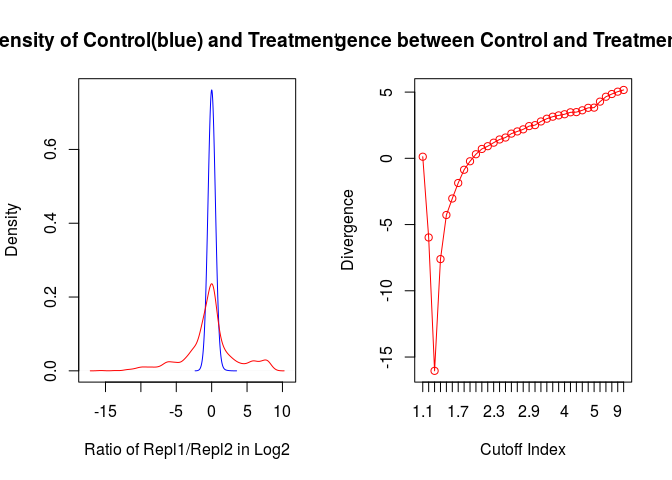• The kernel density plot shows the distribution of logarithm ratios in the control-control dataset and case-control dataset. In general, the control- control distribution should reflects the system noise while the case-control will contains real DEGs and system noises.

• Instead of using all control and case samples, the user could specify a small
sample and perform a pilot study. This is extremely useful if the users are only interested on a small subset of samples.

  fci=find.fci.targets(fci, c(1,2), 5, filename)
## Control-Control Used : [ 1 2 ] & Control-Case Used : [ 1 5 ];  Fold_Cutoff= 1.3 ; Num_Of_DEGs= 820 ; Divergence= 0.00056408
## Control-Control Used : [ 1 2 ] & Control-Case Used : [ 2 5 ];  Fold_Cutoff= 1.3 ; Num_Of_DEGs= 819 ; Divergence= 0.00010632

## 3.5 Alternative function to find DEGs

• Using the same microarray dataset, we could run fCI with a single function call. However, the internal runtime variables and fci object will disappear after the function returns.
if(file.exists(filename)){
Diff.Expr.Genes=fCI.call.by.index(c(1,2,3), c(4,5,6), filename)
}
## Control-Control Used : [ 1 2 ] & Control-Case Used : [ 1 4 ];  Fold_Cutoff= 1.3 ; Num_Of_DEGs= 821 ; Divergence= 0.00015769
## Control-Control Used : [ 1 2 ] & Control-Case Used : [ 2 4 ];  Fold_Cutoff= 1.3 ; Num_Of_DEGs= 811 ; Divergence= 0.00069831
## Control-Control Used : [ 1 2 ] & Control-Case Used : [ 3 4 ];  Fold_Cutoff= 1.3 ; Num_Of_DEGs= 813 ; Divergence= 0.00021425
## Control-Control Used : [ 1 2 ] & Control-Case Used : [ 1 5 ];  Fold_Cutoff= 1.3 ; Num_Of_DEGs= 820 ; Divergence= 0.00056408
## Control-Control Used : [ 1 2 ] & Control-Case Used : [ 2 5 ];  Fold_Cutoff= 1.3 ; Num_Of_DEGs= 819 ; Divergence= 0.00010632
## Control-Control Used : [ 1 2 ] & Control-Case Used : [ 3 5 ];  Fold_Cutoff= 1.3 ; Num_Of_DEGs= 820 ; Divergence= 0.00057505
## Control-Control Used : [ 1 2 ] & Control-Case Used : [ 1 6 ];  Fold_Cutoff= 1.3 ; Num_Of_DEGs= 823 ; Divergence= 0.00023359
## Control-Control Used : [ 1 2 ] & Control-Case Used : [ 2 6 ];  Fold_Cutoff= 1.3 ; Num_Of_DEGs= 813 ; Divergence= 0.00113844
## Control-Control Used : [ 1 2 ] & Control-Case Used : [ 3 6 ];  Fold_Cutoff= 1.3 ; Num_Of_DEGs= 813 ; Divergence= 0.00036187
## Control-Control Used : [ 1 3 ] & Control-Case Used : [ 1 4 ];  Fold_Cutoff= 1.3 ; Num_Of_DEGs= 821 ; Divergence= 1.764e-05
## Control-Control Used : [ 1 3 ] & Control-Case Used : [ 2 4 ];  Fold_Cutoff= 1.3 ; Num_Of_DEGs= 811 ; Divergence= 0.00014706
## Control-Control Used : [ 1 3 ] & Control-Case Used : [ 3 4 ];  Fold_Cutoff= 1.3 ; Num_Of_DEGs= 813 ; Divergence= 1.477e-05
## Control-Control Used : [ 1 3 ] & Control-Case Used : [ 1 5 ];  Fold_Cutoff= 1.3 ; Num_Of_DEGs= 820 ; Divergence= 0.00014493
## Control-Control Used : [ 1 3 ] & Control-Case Used : [ 2 5 ];  Fold_Cutoff= 1.3 ; Num_Of_DEGs= 819 ; Divergence= 2.89e-06
## Control-Control Used : [ 1 3 ] & Control-Case Used : [ 3 5 ];  Fold_Cutoff= 1.3 ; Num_Of_DEGs= 820 ; Divergence= 0.00012726
## Control-Control Used : [ 1 3 ] & Control-Case Used : [ 1 6 ];  Fold_Cutoff= 1.3 ; Num_Of_DEGs= 823 ; Divergence= 5.034e-05
## Control-Control Used : [ 1 3 ] & Control-Case Used : [ 2 6 ];  Fold_Cutoff= 1.3 ; Num_Of_DEGs= 813 ; Divergence= 0.00024359
## Control-Control Used : [ 1 3 ] & Control-Case Used : [ 3 6 ];  Fold_Cutoff= 1.3 ; Num_Of_DEGs= 813 ; Divergence= 3.525e-05
## Control-Control Used : [ 2 3 ] & Control-Case Used : [ 1 4 ];  Fold_Cutoff= 1.3 ; Num_Of_DEGs= 821 ; Divergence= 2e-08
## Control-Control Used : [ 2 3 ] & Control-Case Used : [ 2 4 ];  Fold_Cutoff= 1.3 ; Num_Of_DEGs= 811 ; Divergence= 1.509e-05
## Control-Control Used : [ 2 3 ] & Control-Case Used : [ 3 4 ];  Fold_Cutoff= 1.3 ; Num_Of_DEGs= 813 ; Divergence= 2.82e-06
## Control-Control Used : [ 2 3 ] & Control-Case Used : [ 1 5 ];  Fold_Cutoff= 1.3 ; Num_Of_DEGs= 820 ; Divergence= 8.66e-06
## Control-Control Used : [ 2 3 ] & Control-Case Used : [ 2 5 ];  Fold_Cutoff= 1.3 ; Num_Of_DEGs= 819 ; Divergence= 2.82e-06
## Control-Control Used : [ 2 3 ] & Control-Case Used : [ 3 5 ];  Fold_Cutoff= 1.3 ; Num_Of_DEGs= 820 ; Divergence= 8.36e-06
## Control-Control Used : [ 2 3 ] & Control-Case Used : [ 1 6 ];  Fold_Cutoff= 1.3 ; Num_Of_DEGs= 823 ; Divergence= 5.8e-07
## Control-Control Used : [ 2 3 ] & Control-Case Used : [ 2 6 ];  Fold_Cutoff= 1.3 ; Num_Of_DEGs= 813 ; Divergence= 6.742e-05
## Control-Control Used : [ 2 3 ] & Control-Case Used : [ 3 6 ];  Fold_Cutoff= 1.3 ; Num_Of_DEGs= 813 ; Divergence= 1.48e-05
##   DEG_Names Mean_Control Mean_Case Log2_FC fCI_Prob_Score
## 1         1        0.009     0.002   -2.17              1
## 2        10        0.672     0.091  -2.885              1
## 3       100        1.266     0.399  -1.666              1
## 4      1000        0.239     0.456   0.932              1
## 5      1001        0.136     0.357   1.392              1
## 6      1002        0.004     0.014   1.807              1

## 3.6 Testing fCI on a randomly generated simulated dataset

• In this example, we generated a simulated random dataset with 3 control replicates (column 1 through 3) and 3 case replicates (columns 4 through 6).
fci.data=data.frame(matrix(sample(3:100, 1043*6, replace=TRUE), 1043,6))

### 3.6.1 Finding Differentially Expressed Genes (no DEGs in this case):

• To identify differentially expressed genes using the above simulated data:
 library(fCI)
fci=new("NPCI")
targets=find.fci.targets(fci, c(1,2,3), c(4,5,6), fci.data)
Diff.Expr.Genes=show.targets(targets)
##  "No differentially expressed genes are found!"
 head(Diff.Expr.Genes)
## NULL
 figures(targets)
##  25.10035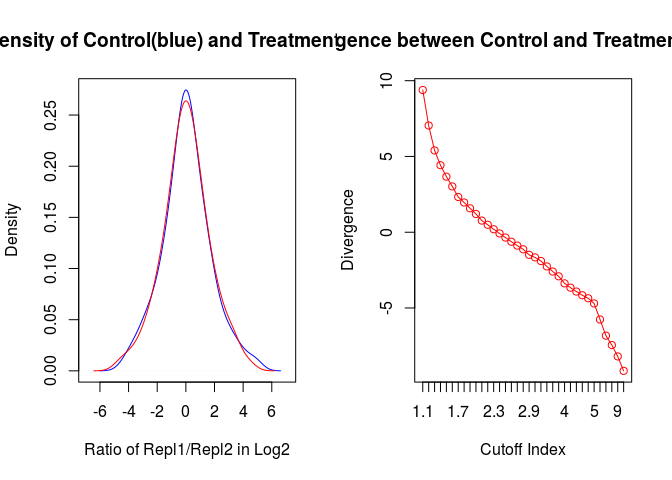• fCI didn’t find a local minimum divergence under the given cutoff fold changes. This confirms that there is indeed no differentially expressed genes.

• This analysis strongly proved that fCI is able to distinguish real DEGs from system noise. If the distribution of case-control didn’t show obivous deviation from control-control, no DEGs will be reported.

# 4 Multi-dimensional (i.e.Pproteogenomics data) fCI analysis

• If the dataset contains multiple control replicates and case replicates, the users don’t need to form the these combinations and perform fCI individually. Instead, users could invoke fci on a top-level function that will automatically perform the analysis on given control replicate column ids and case/experimental column ids.

Formation of empirical & experimental distributions on integrated and/or multidimensional (i.e. time course data). In this example, gene expression values are recorded at c dimensions (c=2 in this figure) with m replicates at each condition from a total of n genes. The ratio of the chosen fCI control-control (or control-case) on 2-dimensional measurements will undergo logarithm transformation and normalization for the analysis. If the pathological or experimental condition causes a number of genes to be up-regulated or down-regulated, a wider distribution which can be described by kernel density distribution (indicated by the 3D ellipse in red) compared to the control-control empirical null distribution (indicated by the 3D ellipse in blue) will be observed. fCI then gradually removes the genes from both tails (representing genes having larger fold changes) from both dimensions using the Hellinger Divergence or Cross Entropy estimation (see methods and materials) until the remaining case-control distribution is very similar or identical to the empirical null distribution, as indicated by the kern density distribution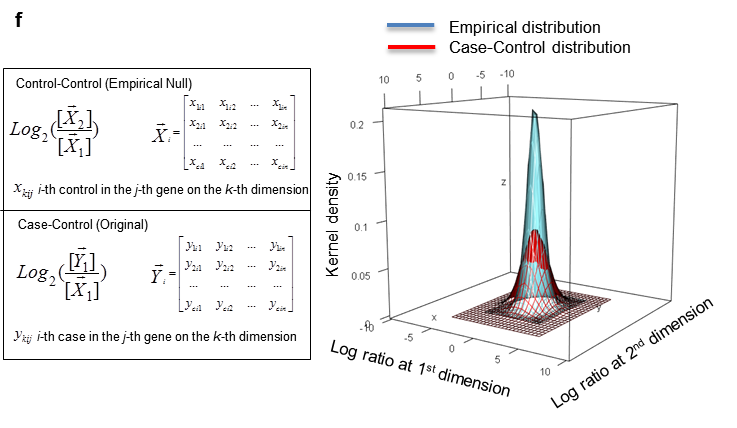## 4.1 Example of integrated proteogeonomics analysis

• Given a dataset with gene expression measured in control, treatment-1 and treatment-2 in both proteomics and transcriptomics data. If the user want to see the co-regulated genes in treatment-1 with respect to control in both proteomics and transcriptomics data. The users just need to indicate the control and experimental samples for both data type respective. For example
fci=new("NPCI")
filename2=paste(pkg.path, "/extdata/proteoGenomics.txt", sep="")
if(file.exists(filename2)){
targets=find.fci.targets(fci, list(1:2, 7:8), list(5:6, 11:12),
filename2)
Diff.Expr.Genes=show.targets(targets)
}
## Control-Control Used :  1 2 7 8  & Control-Case Used :  1 5 7 11  ; Fold_Cutoff= NA ; Num_Of_DEGs= NA ; Divergence= NA
## Control-Control Used :  1 2 7 8  & Control-Case Used :  2 5 7 11  ; Fold_Cutoff= 2.3 ; Num_Of_DEGs= 368 ; Divergence= 0.1451654
## Control-Control Used :  1 2 7 8  & Control-Case Used :  1 6 7 11  ; Fold_Cutoff= 2 ; Num_Of_DEGs= 562 ; Divergence= 0.1187661
## Control-Control Used :  1 2 7 8  & Control-Case Used :  2 6 7 11  ; Fold_Cutoff= 2.3 ; Num_Of_DEGs= 443 ; Divergence= 0.1257171
## Control-Control Used :  1 2 7 8  & Control-Case Used :  1 5 8 11  ; Fold_Cutoff= 2.1 ; Num_Of_DEGs= 548 ; Divergence= 0.1141433
## Control-Control Used :  1 2 7 8  & Control-Case Used :  2 5 8 11  ; Fold_Cutoff= 2.1 ; Num_Of_DEGs= 379 ; Divergence= 0.05388457
## Control-Control Used :  1 2 7 8  & Control-Case Used :  1 6 8 11  ; Fold_Cutoff= 2.2 ; Num_Of_DEGs= 335 ; Divergence= 0.05452131
## Control-Control Used :  1 2 7 8  & Control-Case Used :  2 6 8 11  ; Fold_Cutoff= 2.2 ; Num_Of_DEGs= 421 ; Divergence= 0.04895037
## Control-Control Used :  1 2 7 8  & Control-Case Used :  1 5 7 12  ; Fold_Cutoff= 2.2 ; Num_Of_DEGs= 410 ; Divergence= 0.04585534
## Control-Control Used :  1 2 7 8  & Control-Case Used :  2 5 7 12  ; Fold_Cutoff= 2.2 ; Num_Of_DEGs= 384 ; Divergence= 0.1112904
## Control-Control Used :  1 2 7 8  & Control-Case Used :  1 6 7 12  ; Fold_Cutoff= 2.1 ; Num_Of_DEGs= 456 ; Divergence= 0.09829568
## Control-Control Used :  1 2 7 8  & Control-Case Used :  2 6 7 12  ; Fold_Cutoff= 2.1 ; Num_Of_DEGs= 534 ; Divergence= 0.09129074
## Control-Control Used :  1 2 7 8  & Control-Case Used :  1 5 8 12  ; Fold_Cutoff= 2.2 ; Num_Of_DEGs= 459 ; Divergence= 0.08475181
## Control-Control Used :  1 2 7 8  & Control-Case Used :  2 5 8 12  ; Fold_Cutoff= 2.3 ; Num_Of_DEGs= 279 ; Divergence= 0.03935756
## Control-Control Used :  1 2 7 8  & Control-Case Used :  1 6 8 12  ; Fold_Cutoff= 2.3 ; Num_Of_DEGs= 291 ; Divergence= 0.04108701
## Control-Control Used :  1 2 7 8  & Control-Case Used :  2 6 8 12  ; Fold_Cutoff= 2.2 ; Num_Of_DEGs= 398 ; Divergence= 0.03296388
## A total of  121  genes were identified as differentially expressed.
##   DEG_Names Mean_Control Mean_Case Log2_FC fCI_Prob_Score
## 1      1005       78.629   193.972   1.303              1
## 2      1008      170.615   112.821  -0.597              1
## 3      1022      233.846   355.442   0.604              1
## 4      1071       15.936    23.164    0.54              1
## 5      1078       43.783    54.039   0.304              1
## 6      1089       11.234    15.302   0.446              1
• In this example, the control ids are a list of two independent sample ids. Column 1 and 2 are samples of control proteomics data, and column 7 and 8 are samples of control transcriptomics data. Similarily, Column 5 and 6 are samples of case proteomics data, and column 11 and 12 are samples of case transcriptomics data.

• In other words, the users simply need to give a list of independent control sample ids, and another list of independent case sample ides during the multidimensional analysis.

• The original proteogenomics dataset are indicated in the following

proteogenomic.data=read.csv(filename2, sep="\t")
head(proteogenomic.data)
##         wt1       wt2     pten1     pten2     rapa1     rapa2 geneName.1
## 1  27.15750  36.40941  21.35104  30.74442  34.16499  30.06127   95.08295
## 2 166.19078 151.25397 194.59178 194.94338 113.53789 186.48307   32.36110
## 3 124.66509 128.62288  65.19185  98.03507  92.05198  45.01040  159.18834
## 4  17.05445  30.42224  34.38357  44.61012  44.12769  32.55465   19.16957
## 5 541.11602 598.39787 422.02691 370.37849 604.04739 604.53736   10.10458
## 6  38.70177  64.03203  44.58399  50.98067  51.27947  52.32889   10.24455
##   geneName.2 RPKM_pten1 RPKM_pten2 RPKM_rapamycin1 RPKM_rapamycin2
## 1  98.130548  92.515797 111.881077      147.422682      141.820048
## 2  23.797951  29.805476  23.125577       30.851613       27.032258
## 3 203.869839 212.395122 252.764772      232.002331      220.420246
## 4  17.001337  20.727699  17.895210       18.702316       20.368886
## 5  15.947510  10.107647  11.918627       17.034985       16.325242
## 6   7.734735   7.780514   6.727742        8.104703        7.508715

## 4.2 Specifying fCI runtime variables

• The users can set a variety of fCI, including the control samples, case samples, the predefined fold change cutoff values, and specifiy whether to center the distribution by kernel density or not.
fci=new("NPCI")
fci=setfCI(fci, 7:8, 11:12, seq(from=1.1,to=3,by=0.1), TRUE)

## 4.3 Use only transcriptomics dataset in the proteogenomics data

• Using the same proteogeonomics data, if the user only want to see the differentially expressed genes of treament-1 in transcriptomics only. The unction call becomes:
if(file.exists(filename2)){
fci=find.fci.targets(fci, 7:8, 11:12, filename2)
}
## Control-Control Used : [ 7 8 ] & Control-Case Used : [ 7 11 ];  Fold_Cutoff= 1.5 ; Num_Of_DEGs= 843 ; Divergence= 9.1e-06
## Control-Control Used : [ 7 8 ] & Control-Case Used : [ 8 11 ];  Fold_Cutoff= 1.5 ; Num_Of_DEGs= 644 ; Divergence= 2e-08
## Control-Control Used : [ 7 8 ] & Control-Case Used : [ 7 12 ];  Fold_Cutoff= 1.5 ; Num_Of_DEGs= 783 ; Divergence= 6.85e-06
## Control-Control Used : [ 7 8 ] & Control-Case Used : [ 8 12 ];  Fold_Cutoff= 1.5 ; Num_Of_DEGs= 581 ; Divergence= 0
## A total of  550  genes were identified as differentially expressed.
##   DEG_Names Mean_Control Mean_Case Log2_FC fCI_Prob_Score
## 1         1       86.532   130.509   0.593              1
## 2       100        4.452    17.083    1.94              1
## 3      1000        6.483    15.307   1.239              1
## 4      1001        3.136     8.953   1.513              1
## 5      1002        1.732     2.405   0.474              1
## 6      1005        6.368    11.626   0.868              1
figures(fci)
##  11.37859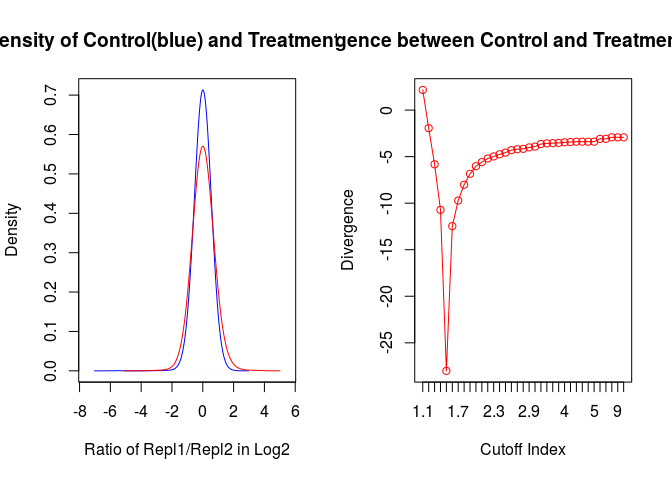# 5 Theory behind fCI

Our method considers transcriptomic (e.g. RPKM values from mapped reads of RNA-Seq experiment) and/or proteomic (e.g. protein peak intensities from TMT LC-MS/MS) data from two biological conditions (e.g. mutant and wild-type or case and control). The goal is to identify the set of genes whose RNA and/or protein levels are significantly changed in the case compared to the control.

In the basic scenario, we require each condition to have two replicates (e.g., RNA, protein or integrated RNA & protein expression data). To identify a set of DEGs in the case samples, the fCI method compares the similarity between the distribution of the case-control ratios (subject to logarithm transformation), denoted P, and similarly the control-control ratios (the empirical null), denoted Q (see Fig 1.c and Supplementary Pseudocode). By construction, Q represents the empirical biological noise, i.e. the ratios from repeated measurements of the same sample. Under mild assumptions, the Almost Sure Central Limit Theorem ensures that P and Q will converge to a univariate/multivariate normal for large sample sizes.

Similarly, we could also construct distributions of P and Q from integrated/multi-dimensional data. In the simplest scenario of a time-course study consisting of two case and control replicates recorded at two time points, the empirical distribution P will be a matrix of two column vectors representing the technical noises, and Q will be a second matrix with case-control ratios, both measured at two time points respectively.

To identify DEGs, we consider the difference between the distributions P and Q as quantified by the f-divergence. The f-divergence is a generalization of the Kullback-Leibler divergence, the Hellinger distance, the total variation distance and many other ways of comparing two distributions based on the odds ratio. Currently, we have implemented two different instances of f-divergence, but it is straightforward to extend the fCI code by adding additional divergences.

The Hellinger distance, H, is one of the most widely used metrics for quantifying the distance between two distributions. The Hellinger distance has many advantageous properties such as being nonnegative, convex, monotone, and symmetric (24, 25)(22, 23). To calculate the Hellinger distance, we first use the Maximum Likelihood Estimate(MLE) to obtain the parameters of the distributions P and Q assuming Gaussian distributions. The distance between two Gaussian distributions becomes: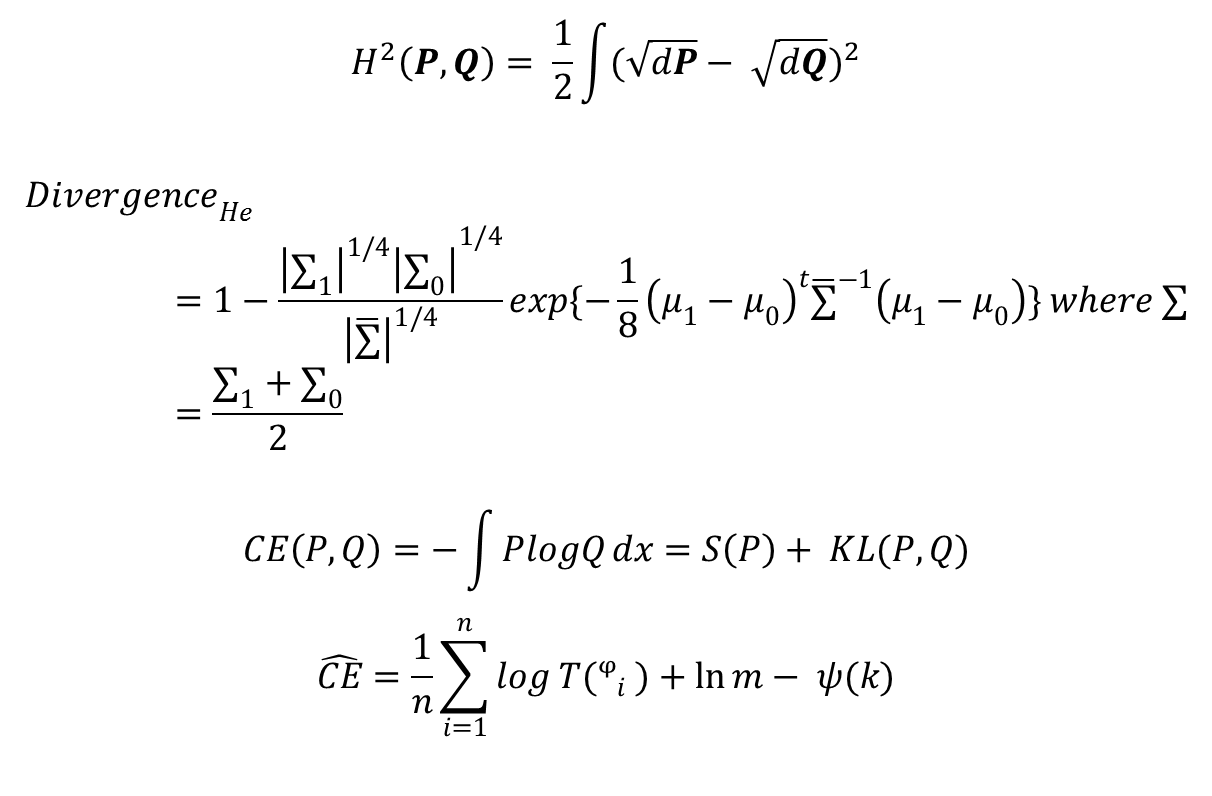If we divide the case-control ratio data into differential and non-differential genes, the remaining non-differential genes (upon the removal of DEGs) from the case-control data should be drawn from the same distribution as the empirical null (7). Therefore, the divergence will be at a global minimum close to 0.

When multiple biological/technical replicates are considered, the control-control ratio and case-control ratio can be formed in pair by mathematical combinations (see Fig 1.b). Otherwise, if replicates are not available for control data, P and Q will be the direct logarithm-transformed distribution of the original gene expression. fCI uses Hellinger distance by default. Empirically, we have found that the cross entropy approach provides more conservative results compared to the Hellinger distance.